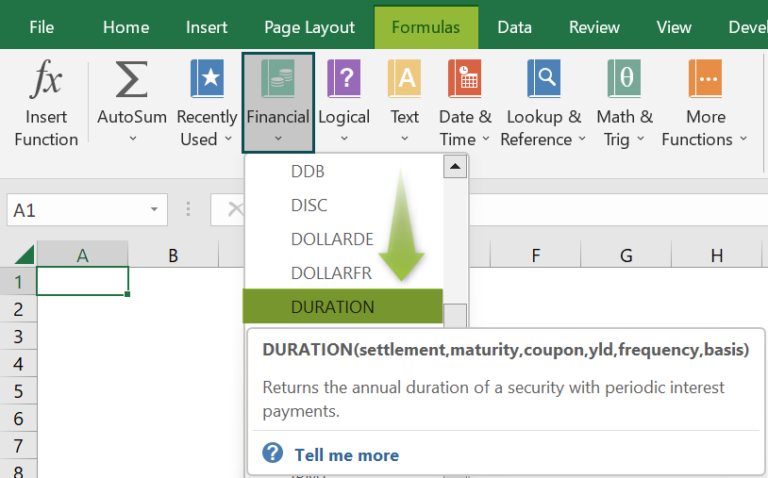# DURATION Excel FunctionArticle byExcelMojo Team## What Is DURATION In Excel?

The DURATION Excel function calculates the bond’s annual duration to pay the loan interest periodically. The cash inflow depends on the coupon and the maturity value at the end. The calculation is also known as Macaulay Duration. The DURATION function in Excel is an inbuilt financial function, so we can insert the formula from the “Function Library” or enter it in the worksheet directly.

For example, the image below depicts the Description in column A and Values in column B. We will apply the DURATION Excel function to calculate the output.

Select cell B4, enter the formula =DURATION(B2,B3,2%,3%,4), and press the “Enter” key.

The result is ‘4.76353017’, as shown above.

###### Key Takeaways
• The DURATION Excel function calculates the Macaulay Duration for an assumed par value of \$100.
• The DURATION may be number of days, months, or years between two dates. However, we use the function to calculate the annual duration of the bond.
• The DURATION function is used to predict future cash flows of investments in financial modelling.
• The Excel DURATION function also helps the users to reduce the impact of the rate of interest that may change their net worth.

### DURATION() Excel Formula

The syntax of the DURATION Excel formula is,

The arguments of the DURATION Excel formula are,

• settlement: It is a mandatory argument. It is the security’s settlement date, or the day the coupon is purchased.
• maturity: It is a mandatory argument. It is the security’s maturity date or the date on which the coupon expires.
• coupon: It is a mandatory argument. It is the security’s coupon rate.
• yld: It is a mandatory argument. The security’s annual yield.
• frequency: It is a mandatory argument. It is the number of coupon payments per year.
• basis: It is an optional argument. It is the type of day count basis to be used.

### How To Use DURATION Excel Function?

We can use the DURATION function in 2 ways, namely,

1. Access from the Excel ribbon.
2. Enter in the worksheet manually.

#### Method #1 – Access from the Excel ribbon

Choose an empty cell for the output 🡪 select the “Formulas” tab 🡪 go to the “Function Library” group 🡪 click the “Financial” option drop-down 🡪 select the “DURATION” function, as shown below.

The “Function Arguments” window appears. Enter the arguments in the “Settlement”, “Maturity”, “Coupon”, “Yld”, “Frequency”, and “basis” fields 🡪 click “OK”, as shown below.

#### Method #2 – Enter in the worksheet manually

1. Select an empty cell for the output.
2. Type =DURATION( in the selected cell. [Alternatively, type =D or =DU and double-click the DURATION function from the list of suggestions shown by Excel.]
3. Enter the arguments as cell values or cell references and close the brackets.
4. Press the “Enter” key.

Let us understand this function with a basic example.

The succeeding example depicts the values of the investment made by Mr. Ron. We will calculate the output using DURATION Excel function.

In the table, the data is,

• Column A contains the Description.
• Column B contains the Value.

The steps to calculate the duration using DURATION function are,

1. Select cell B8, and enter the formula =DURATION(B4, i.e., the settlement date value.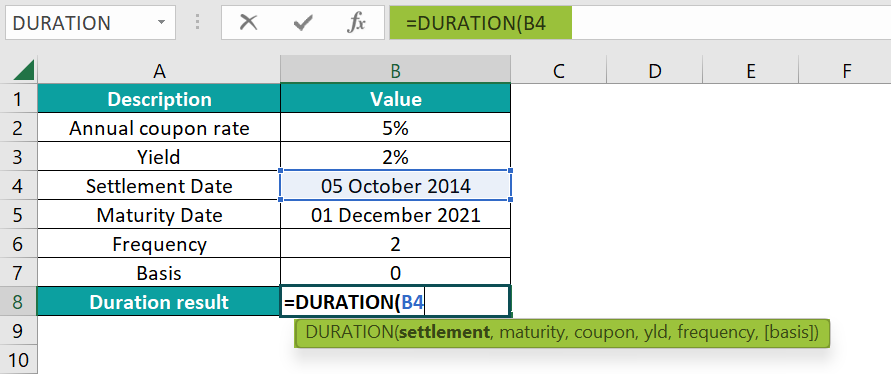2. Enter the value of ‘maturity’ as B5. The formula now is =DURATION(B4,B5,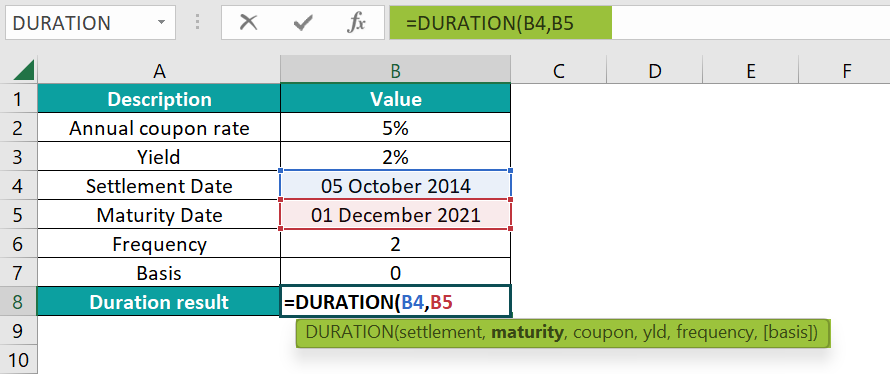3. Enter the value of ‘coupon’ as B2. The formula now is =DURATION(B4,B5,B2,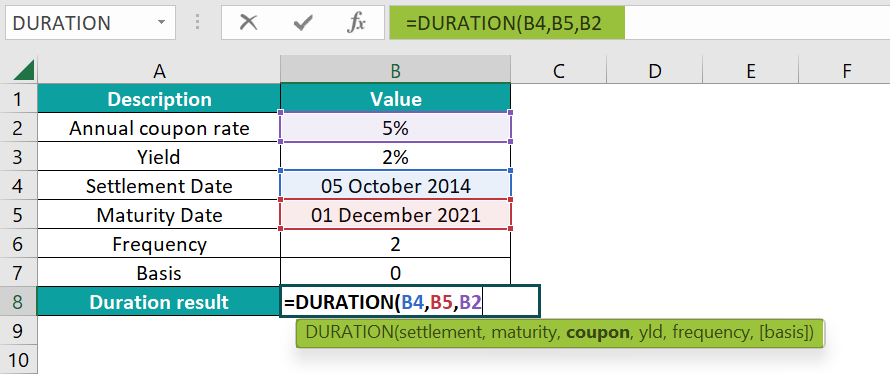4. Enter the value of ‘yld’ as B3. The formula now is =DURATION(B4,B5,B2,B3,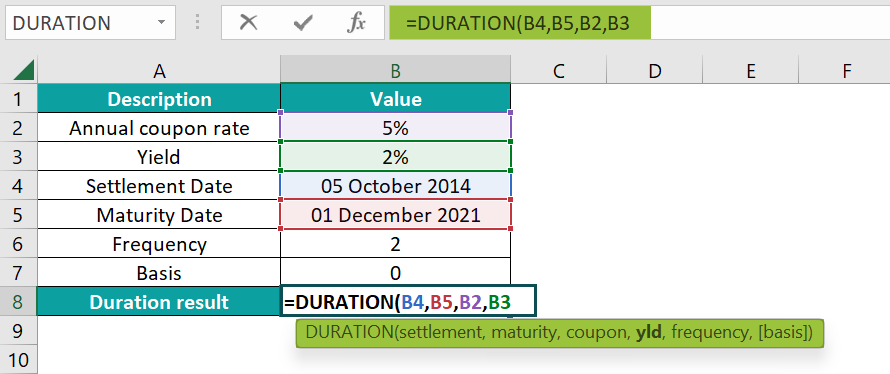5. Enter the value of ‘frequency’ as B6. The formula now is =DURATION(B4,B5,B2,B3,B6,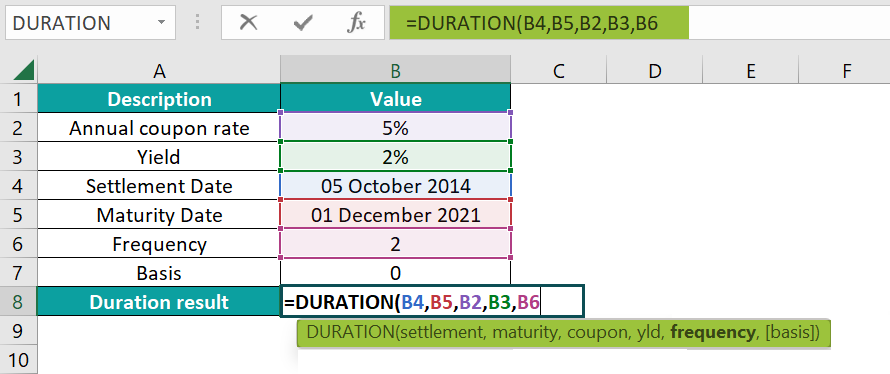6. Enter the value of ‘basis’ as B7, and close the brackets. The complete formula is =DURATION(B2,B3,2%,3%,4)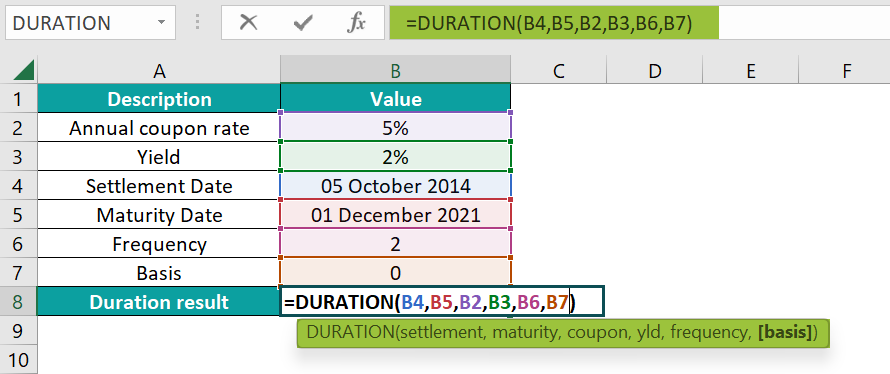7. Press the “Enter” key. The result is “6.12459847”, as shown below.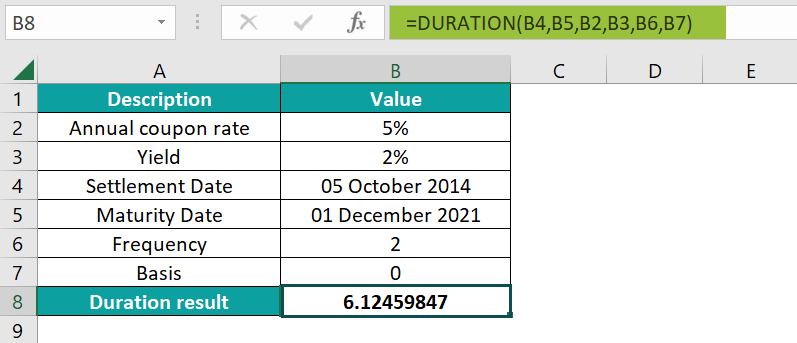### Examples

We will understand some advanced scenarios using the DURATION function examples.

#### Example #1

The succeeding example depicts the values of the investment made by Mrs. Atlenta. We will calculate the output using the DURATION Excel function.

In the table, the data is,

• Column A contains the Description.
• Column B contains the Value.

The procedure to calculate the value using the DURATION function is,

Select cell B4, enter the formula =DURATION(B2,B3,6%,1%,2), and press the “Enter” key.

[Note: The value of ‘settlement’ is B2, ‘maturity’ is B3, ‘coupon’ is 6%, ‘yld’ is 1%, and ‘frequency’ is 2.]

The result is “0.919113647”, as shown above.

#### Example #2

The succeeding example depicts the investment values. We will calculate the “#NUM!” error using the DURATION Excel function.

In the table, the data is,

• Column A contains the Description.
• Column B contains the Value.

The procedure to calculate the value using the DURATION function is,

Select cell B4, enter the formula =DURATION(B2,B3,9%,3%,1), and press the “Enter” key.

[Note: The value of ‘settlement’ is B2, ‘maturity’ is B3, ‘coupon’ is 9%, ‘yld’ is 3%, and ‘frequency’ is 1.]

The result is a “#NUM!” error, as shown above.

#### Example #3

The succeeding example depicts the investment values. We will calculate the “#VALUE!” error using the DURATION Excel function.

In the table, the data is,

• Column A contains the Description.
• Column B contains the Value.

The procedure to calculate the value using the DURATION function is,

Select cell B4, enter the formula =DURATION(B2,B3,2%,3%,4), and press the “Enter” key.

[Note: The value of ‘settlement’ is B2, ‘maturity’ is B3, ‘coupon’ is 2%, ‘yld’ is 3%, and ‘frequency’ is 4.]

The result is a “#VALUE!” error, as shown above.

### Important Things To Note

• The “#NUM!” error occurs when,
• The settlement date is the same as the maturity date.
• The cell values of the arguments are invalid.
• The “#VALUE!” error occurs when,
• If the argument is non-numeric.
• The dates provided are not valid Excel dates.

1. What does the DURATION Excel function mean?

The DURATION Excel function is the Macauley duration We can define Duration as the weighted average of the present value of cash flows, and it is used to measure an investment price’s response to changes in yield argument.

The syntax of the DURATION Excel function is =DURATION(settlement, maturity, coupon, yld, frequency, basis)

2. How to insert the DURATION Excel function?

We can insert the DURATION function in Excel as follows:
1. Select an empty cell for the output.
2. Type =DURATION( in the selected cell. [Alternatively, type =D or =DU and double-click the DURATION function from the list of suggestions shown by Excel.]
3. Enter the arguments as cell values or cell references and close the brackets.
4. Press the “Enter” key.

For example, the image depicts the values of the investment made by XYZ firm, and we will the output using the DURATION Excel function.

In the table, the data is,
● Column A contains the Description.
● Column B contains the Value.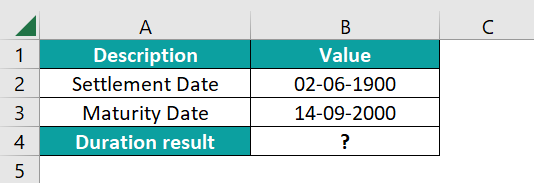The procedure to calculate the value using the DURATION function is,
Select cell B4, enter the formula =DURATION(B2,B3,2%,3%,4), and press the “Enter” key.

[Note: The value of ‘settlement’ is B2, ‘maturity’ is B3, ‘coupon’ is 2%, ‘yld’ is 3%, and ‘frequency’ is 4.]

The result is “33.3606166”, as shown below.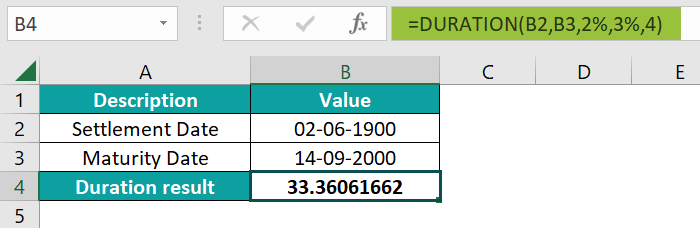3. Where is the DURATION Excel function?

The DURATION Excel function is in the “Formulas” tab, as shown below.

Choose an empty cell for the output 🡪 select the “Formulas” tab 🡪 go to the “Function Library” group 🡪 click the “Financial” option drop-down 🡪 select the “DURATION” function, as shown below.